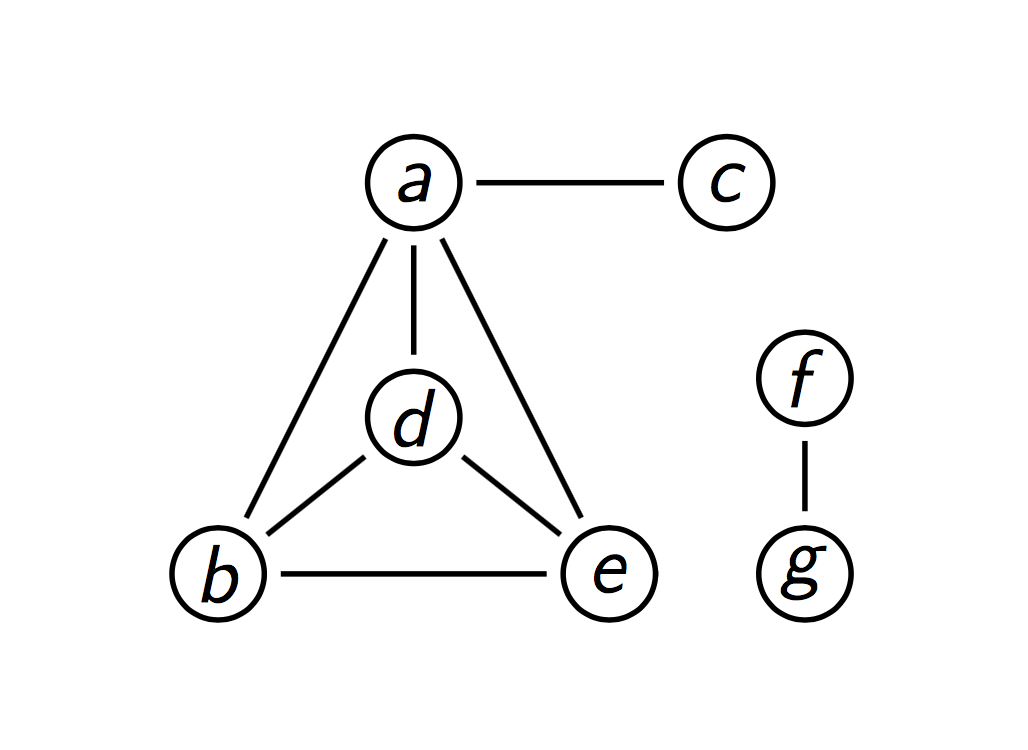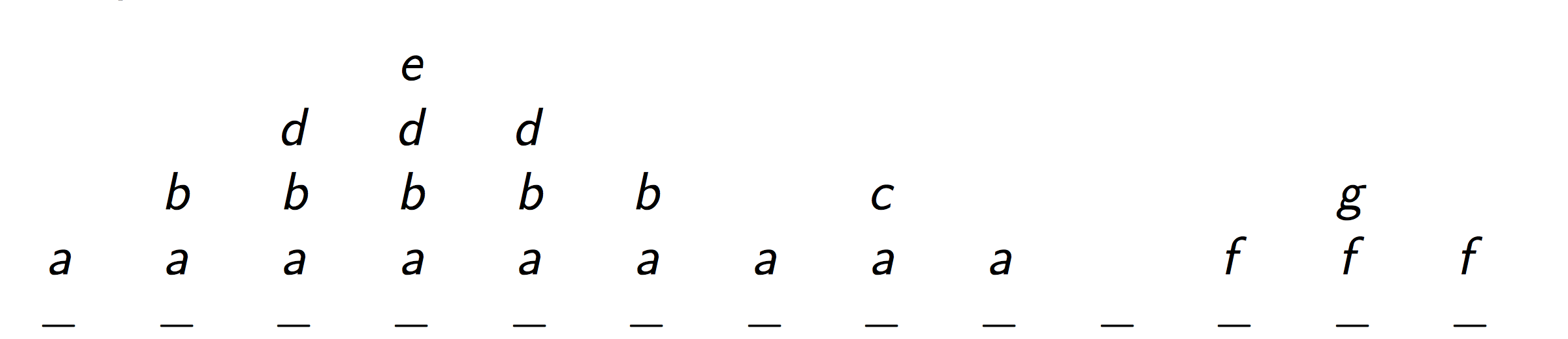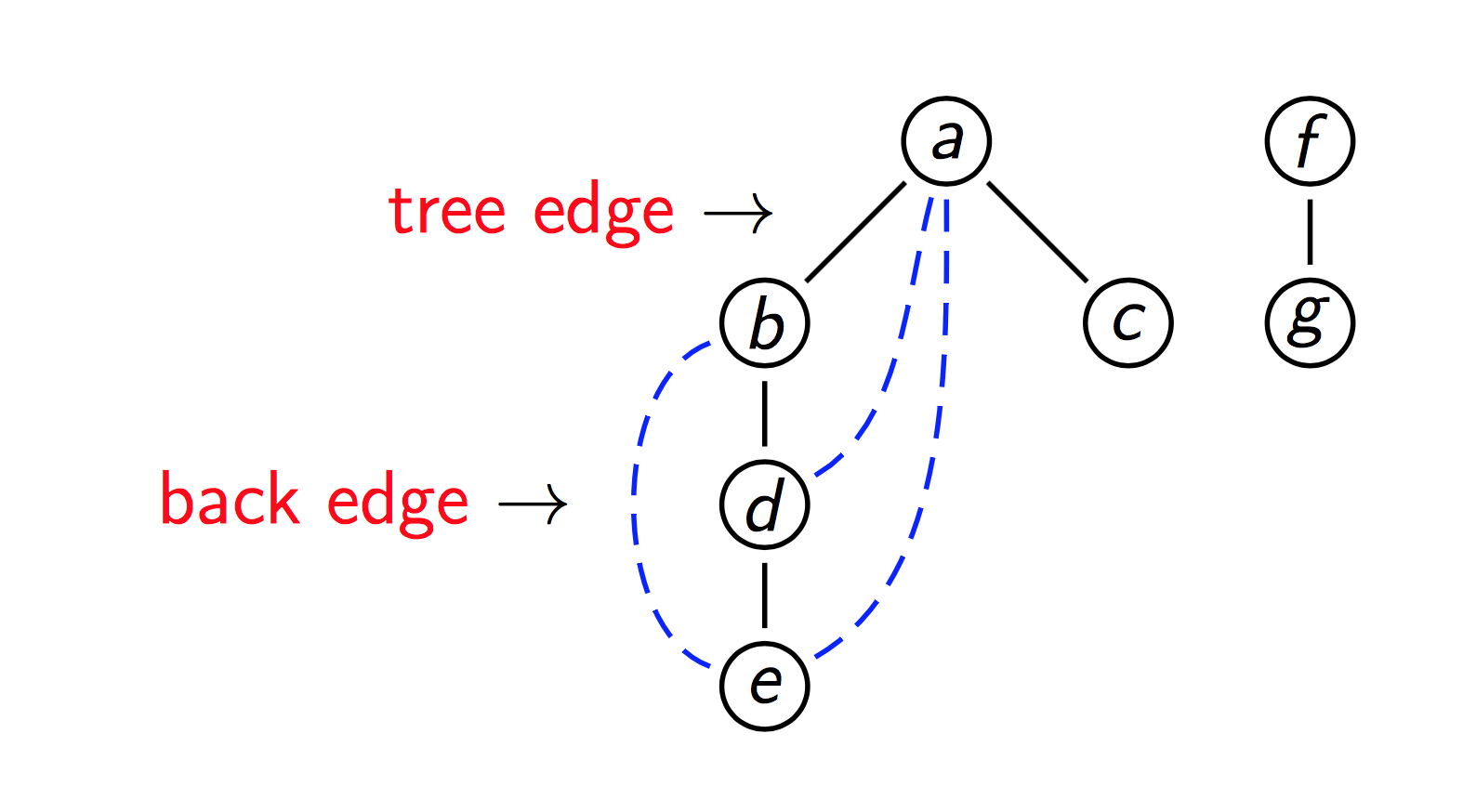# 图——深度优先搜索(Graph - Depth First Search)

Depth-first search(DFS) is an algorithm for traversing or searching tree or graph data structure. One starts at the root(selecting some arbitrary node as the root in the case of a graph) and explores as far as possible along each branch before backtracking.DFS order: a, b, d, e, c, f, g.

We can use a traversal stack to track where we are in overall process.Push order: a, b, d, e, c, f, g.
Pop order: e, d, b, c, a, g, f.

Push nodes into stack when visiting. Pop nodes as all of its neighbours are visited.

Actually, pushing-order gives us node-visiting order which is DFS order. Poping-order gives us backtracking path.

We can draw DFS tree(forest) to depict DF-traversal.function DFS(<V, E>)
mark each node in V with 0
count ⟵ 0
for each v in V do
if v is marked with 0 then
DFSExplore(v)

function DFSExplore(v)
count ⟵ count + 1
mark v with count
for each edge (v, w) do
if w is marked with 0 then
DFSExplore(w)

We can implement marking nodes by maintaining an array mark[] indexed by V. For example, “mark v with count” = “mark[v] = count”. The value of count represents the order number visited. For instance, “mark[v] = 3”. It means node v is the third node visited.

If we use adjacency matrix to represent a graph. The time for finding each edge (v, w) is |V|^2. Hence the complexity of graph traversal is (|V| + |V|^2), which is Θ(|V|^2).

If using adjacency list, for each v we traverse the list adj[v] to find all its neighbour. It will take |E| times. overall, its time complexity is Θ(|V| + |E|).

Based on the algorithm, answer those following questions.
1. How to decide whether a graph is connected?
2. how to decide whether a graph has a cycle?
3. In terms of DFS tree(forest), how can we tell if we have traversed a dag.

DFS is a great tree traversal and searching technique.

Ask yourself, what practical problems can we solve using DFS? Give specific example.

Welcome questions always and forever.©️2019 CSDN 皮肤主题: 编程工作室 设计师: CSDN官方博客